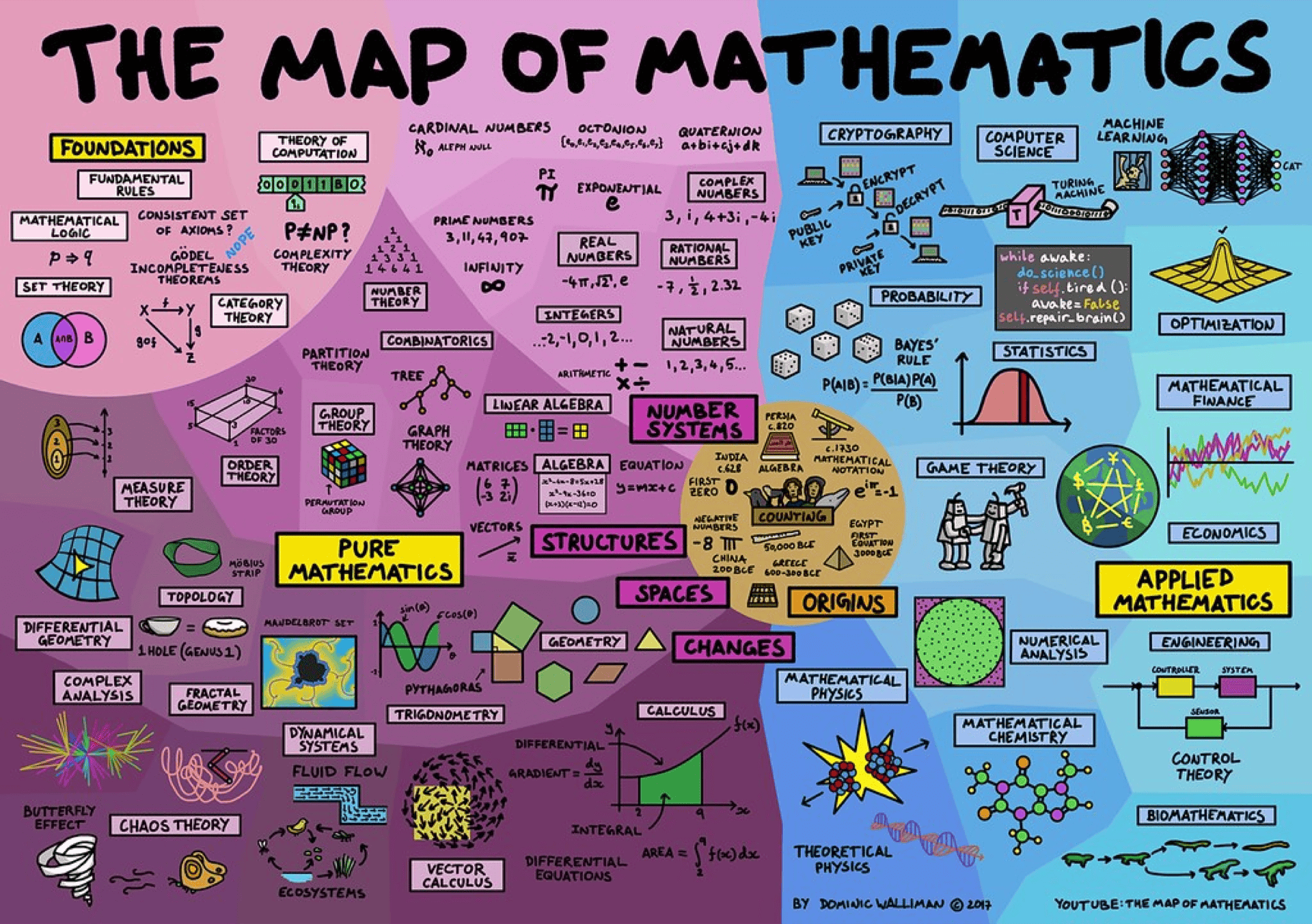# 🔢 Math MOC §

Mathematics is a language to express yourself. Master mathematics and you will be a step closer to unlocking your thinking. I really like the Rigor that math provides, which is so useful in applications such as ML.Mathematics is useful in so many areas because it is abstract: the same good idea can unlock the problems of control engineers, civil engineers, physicists, social scientists, and mathematicians because the idea has been abstracted from a particular setting. One technique solves many problems because someone has established a theory of how to deal with these kinds of problems.

• Definitions are the way we try to capture important ideas, and
• theorems are how we summarize useful general facts about the kind of problems we are studying.
• Proofs not only show us that a statement is true; they can help us understand the statement, give us practice using important ideas, and make it easier to learn a given subject. In particular, proofs show us how ideas are tied together, so we do not have to memorize too many disconnected facts

Is math invented or discovered?

Is anything really invented? Check out this video .